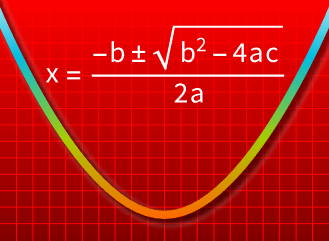# Algebraic Computation

Version 12 of the Wolfram Language extends its already strong capabilities in algebraic computation with functionality for users ranging from pre-college students to researchers. Improvements span from new functions that manipulate equations step by step to more powerful equation and optimization solvers to equational theorem proving.• Compute the boundary, connected components and other topological properties of solution sets for real polynomial systems. »
• Express large systems naturally and succinctly using vector and matrix variables. »
• Efficiently solve large systems of equations and inequalities with special structure. »
• Solve previously intractable classes of equation and inequality systems. »
• Formulate optimization problems in a more concise and natural way using vector and matrix variables. »
• Solve new classes of exact optimization problems. »
• Solve equations "step by step" using new functions to add, multiply and otherwise combine equations and inequalities. »
• Produce automatic proofs of equational logic theorems. »
• Prove theorems using a built-in collection of axiomatic theories. »
• Present proofs in various formats, including graphs, datasets and notebooks. »